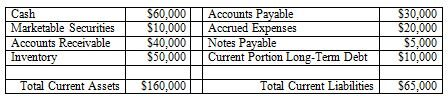## What are Quick Assets?

Quick assets are defined as assets that can quickly be converted to cash. Most typically, quick assets include: cash, accounts receivable, marketable securities, and sometimes (not usually) inventory.

## Quick Assets Example

Let's say that Company XYZ has \$60,000 in cash, \$40,000 in receivables, and \$10,000 in marketable securities. In this example of quick assets, we would say that Company XYZ has \$110,000 in quick assets.

## Why Are Quick Assets Important?

Quick assets are a key part of the quick ratio, a measure of whether – and how well – a company can pay its short-term financial liabilities. Quick ratio is also referred to as the acid-test ratio.

A common rule of thumb is that a quick ratio of 1-to-1 (or greater) means that a company can pay its current liabilities.

## Quick Ratio Formula

The most common formula for quick ratio is as follows:

(Cash + Marketable Securities + Accounts Receivable)/Current Liabilities

### Quick Ratio Formula ExampleUsing the primary quick ratio formula and the information above, we can calculate that XYZ Company’s quick ratio is:

(\$60,000 + \$10,000 + \$40,000)/\$65,000 = 1.692

This means that for every dollar of XYZ Company’s current liabilities, XYZ Company had \$1.69 of very liquid assets to pay those liabilities.